Home MonkeyNotes Printable Notes Digital Library Study Guides Study Smart Parents Tips College Planning Test Prep Fun Zone Help / FAQ How to Cite New Title Request

 CHAPTER 7 : DEFINITE INTEGRAL 7.1 Definition If for any partition of [a, b] the lim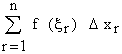when n tends to infinity in such a way that the largest of all the subinterval D x1 , D x2 , ......., D xn tends to zero, exists, then the function which is continuous on [a, b] is said to be integrable over the closed interval [a, b] and it is denoted by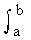f(x) dx. . Let f (x) be any function of x, assumed to be continuous on the closed interval [a , b]. Divide [a , b] into a finite number, say n sub-intervals (not necessarily equal) by means of points x1 , x2 , ......., xn -1 such that a = x0 < x 1 < x 2 ........... < x n -1 < xn = b This is called a partition of [a, b] into n sub intervals. Let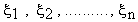be numbers such that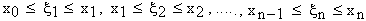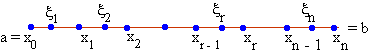Now the subsequent function values at these numbers are f ( x1), f (x2 ), f (x3), ......., f (xn ) respectively. Then the products of each function value and its sub interval length are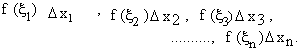Now consider the sum i.e.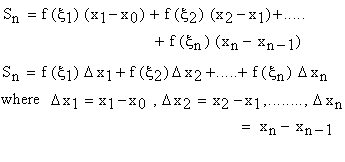This sum is called as "Riemann Sum" which can be positive, negative or zero depending upon the behavior of f (x) on [a, b]. For example If f (x)0 on [a, b] the Sn is a positive real number. If f (x)0 on [a, b] then Sn is a negative real number. Now if the number of these sub intervals increased indefinite, by the lengths of each sub interval would get smaller and smaller i.e. n ® ¥ then Dx ® 0. Under this effect, the limit of this Riemann Sum (Sn ) if exists, then f (x) is said to be integrable on [a, b]. This limit is called the definite integral of f (x) on [a , b] and is denoted byThus the definite integral of f (x) from a to b is defined as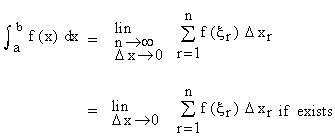Index Chapter 8